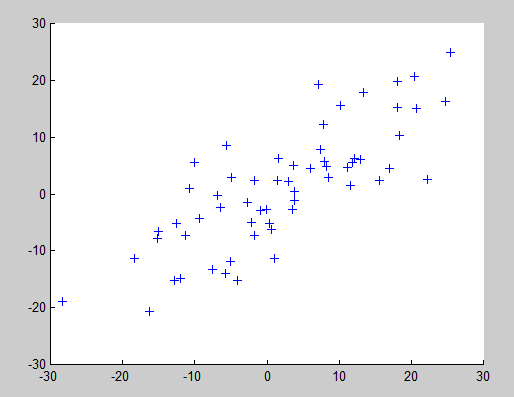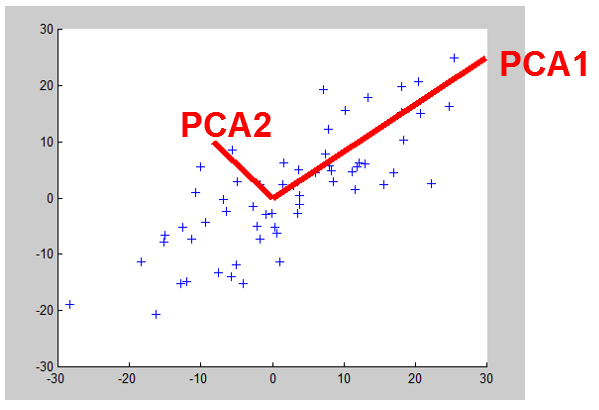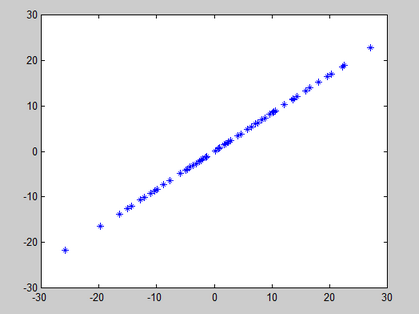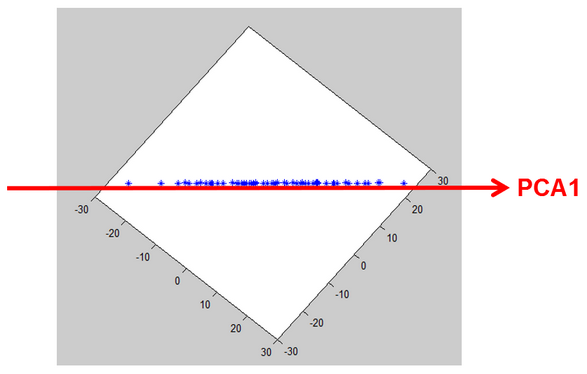﻿ 2. Introduction to the PCA techniques > 2.1. Dimensionality reduction > 2.1.1. A first example: from 2D to 1D

# 2.1.1. A first example: from 2D to 1D

To explain how to use the PCA technique to do « dimentionality reduction », we will start with a small example. We have here many points in 2D (2 dimensions) and we want to reduce the dimension to 1D:(For the census-income database, we have points in 7D and we want to reduce the coordinates in 3D).

The output of the PCA analysis is a set of different perpendicular directions represented in red on the chart below:Let’s project all the blue points of the database on the first PCA axis (PCA1): the projection is illustrated in this chart in green:We obtain a new dataset that is represented here in blue:This new dataset can be “seen” in one dimension along the PCA1 direction:We just reduced the dimension: the 2D points are now in a one-dimensional-space. During the dimensionality reduction, we didn’t lose too much information because the distance between the (same) point “before” and “after” projection is small. This is thus a good dimensionality reduction.